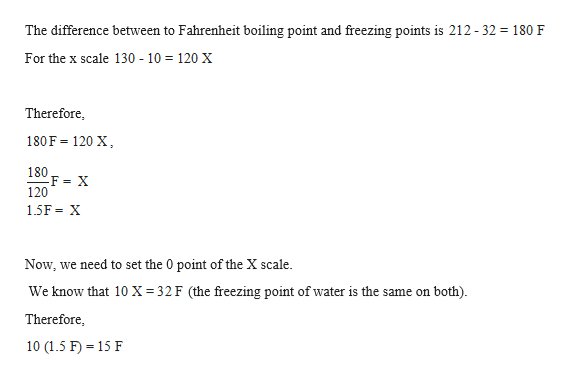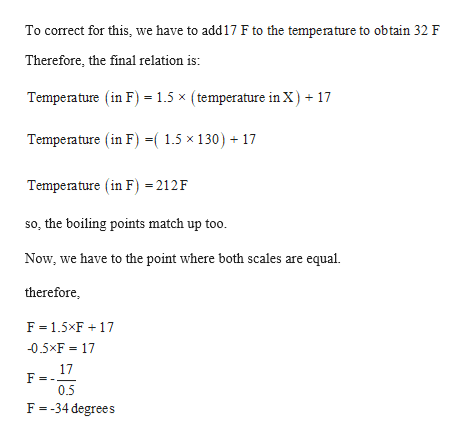# Suppose you design a new thermometer called X thermometer. On X scale, the boiling point is 130 degree X and freezing point of water is 10 degree X. At what temp are readings on the Fahrenheit and X thermometers the same?  Answer supposedly:-34 degrees Fahrenheit How?

Question
39 views

Suppose you design a new thermometer called X thermometer. On X scale, the boiling point is 130 degree X and freezing point of water is 10 degree X. At what temp are readings on the Fahrenheit and X thermometers the same?

How?

check_circle

star
star
star
star
star
1 Rating
Step 1

Given,

The boiling point is 130 degree X and freezing point of water is 10 degree X.help_outlineImage TranscriptioncloseThe difference between to Fahrenheit boiling point and freezing points is 212 - 32 180 F For the x scale 130 10 120 X Therefore, 180F 120 X 180 120 1.5F X Now, we need to set the 0 point of the X scale We know that 10 X 32F (the freezing point of water is the same on both) Therefore 10 (1.5 F) 15 F fullscreen
Step 2

The temperature is...help_outlineImage TranscriptioncloseTo correct for this, we have to add17 F to the temperature to obtain 32 F Therefore, the final relation is Temperature (in F) 1.5 x (temperature in X)17 Temperature (in F) =( 1.5 x 130) + Temperature (in F) =212F so, the boiling points match up too. Now, we have to the point where both scales are equal. therefore F 1.5xF 17 -0.5xF 17 17 F 0.5 F -34 degrees fullscreen

### Want to see the full answer?

See Solution

#### Want to see this answer and more?

Solutions are written by subject experts who are available 24/7. Questions are typically answered within 1 hour.*

See Solution
*Response times may vary by subject and question.
Tagged in

### General Chemistry